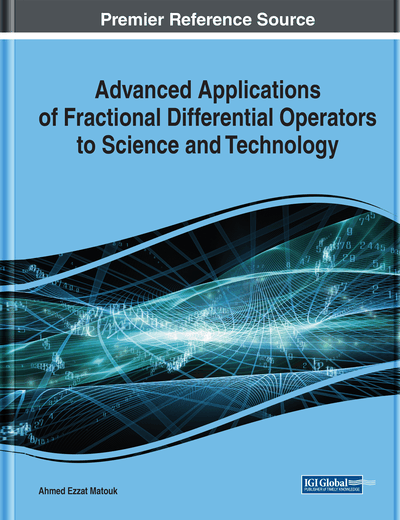# Revisiting Anomalous g-Factors for Charged Leptons in a Fractional Coarse-Grained Approach With Axiomatic Local Metric Derivatives

José Weberszpil (Universidade Federal Rural do Rio de Janeiro, Brazil) and José Abdalla Helayël-Neto (Centro Brasileiro de Pesquisas Físicas, Brazil)
DOI: 10.4018/978-1-7998-3122-8.ch008

## Abstract

This contribution sets out to extend the concept of helicity so as to include it in a fractional scenario with a low-level of fractionality. To accomplish this goal, the authors write down the left- and the right-handed Weyl equations from first principles in this extended framework. Next, by coupling the two different fractional Weyl sectors by means of a mass parameter, they arrive at the fractional version of Dirac's equation, which, whenever coupled to an external electromagnetic field and reduced to the non-relativistic regime, yields a fractional Pauli-type equation. From the latter, they are able to present an explicit expression for the gyromagnetic ratio of charged fermions in terms of the fractionality parameter. They then focus their efforts to relate the coarse-grained property of space-time to fractionality and to the (g-2) anomalies of the different leptonic species. To do this, they build up an axiomatic local metric derivative that exhibits the Mittag-Leffler function as eigenfunction and is valid for low-level fractionality, whenever the order parameter is close to 1.
Chapter Preview
Top

## Introduction

The electron gyromagnetic ratio, ge, is a dimensionless parameter that appears in the spin-orbit interaction and leads to a splitting in the atomic energy levels, giving rise to the so-called fine structure. The ge-factor appears in the Zeeman effect, by means of the electron's magnetic dipole moment, in the description of the interaction of atoms with external magnetic fields.

It is now known that the anomalous magnetic moment (AMM) of the muon is considered to be one of the most promising observables that can unveil effects of some new Physics beyond the Standard Model (Passera et al, 2008). One also claims that the muon's AMM is one of the most precisely measured quantities in Particle Physics, reaching a precision of 0.54ppm (Jegerlehner, & Nyffeler, 2009). This statement is also reinforced in view of the relatively large value of the muon's mass (de Rafael, 2013). The tau-lepton gyromagnetic ratio is not very well-known experimentally and for this reason it does not provide yet a good test of the Standard Model (SM) and for Physics beyond the Standard Model (de Rafael, 2013).

Relativistic quantum mechanics predicts the remarkable result, ge=2. Experimentally, the electron's g-ratio has been measured with high precision and the ”2010 CODATA value” (Mohr et al, 2012) for this parameter is, which is supported by the theoretical calculations of Quantum Electrodynamics (QED), slightly different from that of Dirac's theoretical prevision in the realm of Relativistic Quantum Mechanics (QM). A good review on the introduction and history of the g-ratios, up to 2007, may be found in (Miller et al, 2007). For a non-perturbative approach, the reader could consult (Brodsky et al, 2004). An update of the electron's and muo's g-ratios can be found in (de Rafael, 2013).

In this contribution, we revisit the investigation of the fractional coarse-grained aspects of the electron's anomalous magnetic g-ratio (Weberszpil & Helayël-Neto, 2014) with the recent developed axiomatic local deformed derivative (ALDD) (Weberszpil & Helayël-Neto, 2017a), showing the possibility of mapping its experimental value into some theoretical parameter that accounts for fractionality, so that we take the viewpoint that fractionality could be responsible for the deviation from the ge=2 relativistic quantum-mechanical result. This claim highlights that our motivation to adopt a local deformed operator is more physical than a simple mathematically-motivated extension of classical calculus. This becomes more explicit once we argue that the possible justification for the experimental difference from the theoretical Dirac's value ge=2 is imputed to the complexity of the interactions of the electrons, taken as a pseudo-particle ”dressed” by the interactions and the medium. Here, we look at a dynamical system as an open system that can interact with the environment and we argue that ALDD may become an important tool to study open classical and quantum systems, but considering the limits of low level of fractionality.

The ALDD is a local deformed derivative (LDD) that has the Mittag-Leffler function as an eigenfunction and for which deformed Leibniz’ and the chain rule hold, similarly to the standard calculus, but whenever a low-level of fractionality is considered. The deformed operators here are local.

Our main goal here is to show that it is possible to build up a local formalism that meets certain similarities to those required by a version of fractional calculus (FC) (Jumarie, 2006; Jumarie, 2009a; Jumarie, 2013), in such a way that reconciles the relevant axioms of the approach outlined here and, additionally, it is an approximation valid within a low fracionality limit. In this way, we try to make clear, in short, that the ALDD operators are in fact local and behave as an approximations but, provided that the order of the derivative is very close to one. That is, being local and considered as approximations.

## Complete Chapter List

Search this Book:
Reset